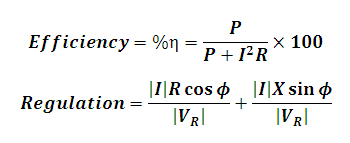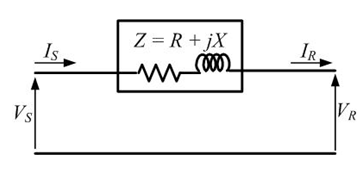# Efficiency and Regulation of Short Transmission Line Calculator

This Calctown Calculator calculates the efficiency and voltage regulation of short transmission lines.

kW
A
ohm
ohm
deg
kV

#### Resultwhere

P = receiving end power

I = transmission line current = Ir(receiving end current) = Is(sending end current)

R = line resistance

X = line reactance

Vs = sending end voltage

Vr = receiving end voltage

φ = receiving end phase angle between current and voltage

η = efficiency